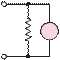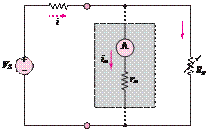### Create an Account

Already have account?

### Forgot Your Password ?

Home / Questions / A circuit that measures the internal resistance of a practical ammeter is shown in Figure ...

# A circuit that measures the internal resistance of a practical ammeter is shown in Figure P274 where RS 50000 Q VS 12 V and Rp is a variable resistor that can be adjusted at will

A circuit that measures the internal resistance of a practical ammeter is shown in Figure P2.74, where R= 50,000 QV= 12 V, and Ris a variable resistor that can be adjusted at will.

a.    Assume that r« 50,000 Q. Estimate the current i.

b.    If the meter displays a current of 150 μA when

R= 15 Q, find the internal resistance of the

I= 120 mA. Find the voltage across R4 with and without the voltmeter in the circuit for the following values:

a. R4 = 100 Q

b.    R4 = 1 kQ

c.    R4 = 10 kQ

d.R4 = 100 kQ

meter r.

IS                                                                                                       120 kÙ           VRS                                                                                                                                                                                                                    4

Figure P2.74Figure P2.77

Jun 19 2020 View more View Less

#### Answer (Solved)Subscribe To Get Solution